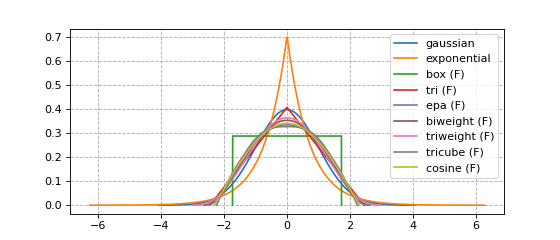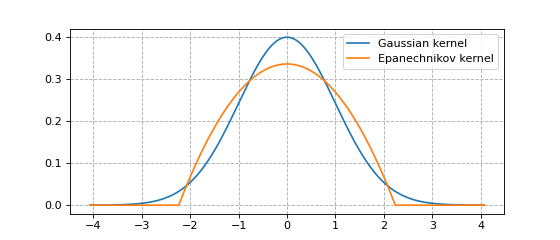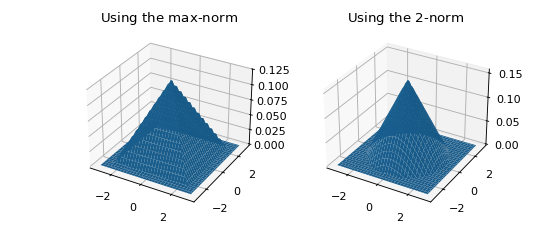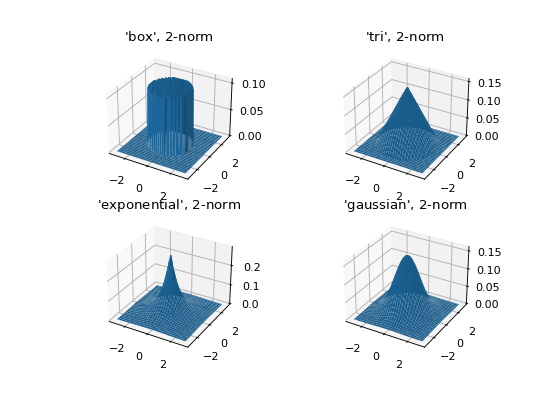# Kernels¶

## Available kernels¶

You are likely reading this because you wonder which kernel functions $$K$$ are available. Every available kernel is shown in the figure below. Kernels with finite support (synonymous with bounded support in these docs) are annotated with an F. A listing of every available option is found in NaiveKDE._available_kernels.keys().

from KDEpy import NaiveKDE

for name, func in NaiveKDE._available_kernels.items():
x, y = NaiveKDE(kernel=name).fit().evaluate()
plt.plot(x, y, label=name + (' (F)' if func.finite_support else ''))

plt.grid(True, ls='--', zorder=-15); plt.legend();## Kernel properties¶

The kernels implemented in KDEpy obey some common properties.

• Normalization: $$\int K(x) \, dx = 1$$.
• Unit variance: $$\operatorname{Var}[K(x)] = 1$$ when the bandwidth $$h$$ is 1. The bandwith of the each kernel equals its standard deviation.
• Symmetry: $$K(-x) = K(x)$$ for every $$x$$.

### Kernels are radial basis functions¶

Symmetry follows from a more general property, namely the fact that every kernel implemented is a radial basis function. A radial basis function is a function which evaluates to the same value whenever the distance from the origin is the same. In other words, it is the composition of a norm $$\left\| \cdot \right\| _p: \mathbb{R}^d \to \mathbb{R}_+$$ and a function $$\kappa: \mathbb{R}_+ \to \mathbb{R}_+$$.

$K(x) = \kappa \left( \left\| x \right\| _p \right)$

Note

If you have high dimensional data with different scales, consider standardizing the data before feeding it to a KDE.

### Kernels may have finite support, or not¶

A given kernel may or may not have finite support. A kernel with finite (or bounded) support is defined on a domain such as $$[-1, 1]$$, while a kernel without finite support is defined on $$(-\infty, \infty)$$.

Below we plot the Guassian kernel and the Epanechnikov kernel.

• The Gaussian kernel does not have finite support.
• The Epanechnikov kernel has finite support.

The reason why kernels are normalized to unit variance is so that bounded and non-bounded kernel functions are more easily compared. In other words, setting bw=1 in the software ensures that the standard deviation $$\sigma = 1$$.

from KDEpy import *

x, y1 = NaiveKDE(kernel='gaussian', bw=1).fit().evaluate()
y2 = NaiveKDE(kernel='epa', bw=1).fit().evaluate(x)
plt.plot(x, y1, label='Gaussian kernel')
plt.plot(x, y2, label='Epanechnikov kernel')
plt.grid(True, ls='--', zorder=-15); plt.legend();## Higher dimensional kernels¶

The one-dimensional example is deceptively simple, since in one dimension every $$p$$-norm is equivalent. In higher dimensions, this is not true. The general $$p$$-norm is a measure of distance in $$\mathbb{R}^d$$, defined by

$\left\| x \right\| _p := \bigg( \sum_{i=1} \left| x_i \right| ^p \bigg) ^{1/p}.$

The three most common $$p$$-norms are

• The Manhattan norm $$\left\| x \right\| _1 = \sum_{i} \left| x_i \right|$$
• The Euclidean norm $$\left\| x \right\| _2 = \sqrt{x_1^2 + x_2^2 + \dots + x_d^2}$$
• The max-norm $$\left\| x \right\| _\infty = \max_{i} \left| x_i \right|$$

In higher dimensions, a norm must be chosen in addition to a kernel. Let $$r := \left\| x \right\| _p$$ be a measure of distance ($$r$$ stands for radius here). The normalization necessary to ensure $$\int \kappa ( \left\| x \right\| _p ) \, dx = 1$$ depends on the value of $$p$$, but symmetry is guaranteed since $$\left\| -x \right\| _p = \left\| x \right\| _p$$. The figure below shows the effect of choosing different norms with the same kernel.

from KDEpy.BaseKDE import BaseKDE
from mpl_toolkits.mplot3d import Axes3D

kernel = BaseKDE._available_kernels['tri']

n = 64
p = np.linspace(-3, 3, num=n)
obs_x_dims = np.array(np.meshgrid(p, p)).T.reshape(-1, 2)

ax = fig.add_subplot(1, 2, 1, projection='3d')
z = kernel(obs_x_dims, norm=np.inf).reshape((n, n))
surf = ax.plot_surface(*np.meshgrid(p, p), z)
ax.set_title('Using the $\max$-norm')

ax = fig.add_subplot(1, 2, 2, projection='3d')
z = kernel(obs_x_dims, norm=2).reshape((n, n))
surf = ax.plot_surface(*np.meshgrid(p, p), z)
ax.set_title('Using the $2$-norm')### Kernel normalization¶

Kernels in any dimension are normalized so that the integral is unity for any $$p$$. To explain how a high-dimensional kernel is normalized, we first examine high dimensional volumes.

Let $$r := \left\| x \right\| _p$$ be the distance from the origin, as measured by some $$p$$-norm. The $$d$$-dimensional volume $$V_d(r)$$ is proportional to $$r^d$$. We will now examine the unit $$d$$-dimensional volume $$V_d := V_d(1)$$.

We integrate over a $$d-1$$ dimensional surface $$S_{d-1}(r)$$ to obtain $$V_{d}$$ using

$V_d = \int_0^1 S_{d-1}(r) \, dr.$

Since a $$d-1$$ dimensional surface is proportional to $$r^{d-1}$$, we write it as $$S_{d-1}(r) = K_{d-1} r^{d-1}$$, where $$K_{d-1}$$ is a constant. Pulling this out of the integral, we are left with

$V_d = K_{d-1} \int_0^1 r^{d-1} \, dr = K_{d-1} / d.$

From this we observe that $$K_{d-1} = V_d \cdot d$$, a fact we’ll use later.

What is the volume of a unit ball $$V_d$$ in the $$p$$ norm in $$d$$ dimensions? Fortunately an analytical expression exists, it’s given by

$V_d(r=1; p) = 2^d \frac{\Gamma \left( 1 + \frac{1}{p} \right)^d}{\Gamma \left(1 + \frac{d}{p} \right)}.$

For more information about this, see for instance the paper by Wang in Literature review, where we have used $$K_{d-1} = V_d \cdot d$$. The equation above reduces to more well-known cases when $$p$$ takes common values, as shown in the table below.

High dimensional volumes
$$p$$ Name Unit volume $$V_d$$
$$1$$ Cross-polytope $$\frac{2^d}{d!}$$
$$2$$ Hypersphere $$\frac{\pi^{d/2}}{\Gamma\left ( \frac{d}{2} + 1 \right )}$$
$$\infty$$ Hypercube $$2^d$$

### Example - normalization¶

We would like to normalize kernel functions in higher dimensions for any norm. To accomplish this, we start with the equation for the volume of a $$d$$-dimensional volume. The equation is

$V_d = K_{d-1} \int_0^1 r^{d-1} \, dr = V_{d} \cdot d \int_0^1 r^{d-1} \, dr.$

The integral of the kernel $$\kappa: \mathbb{R}_+ \to \mathbb{R}_+$$ over the $$d$$-dimensional space is then given by

$V_{d} \cdot d \int_0^1 \kappa(r) \, r^{d-1} \, dr,$

which we can compute. For instance, the linear kernel $$\kappa(r) = (1-r)$$ is normalized by

$V_{d} \cdot d \int_0^1 \left ( 1 - r \right ) r^{d-1} \, dr = V_{d} \cdot d \left ( \frac{1}{d} - \frac{1}{d+1} \right )= V_d \left ( \frac{1}{d+1} \right ).$

The biweight kernel $$\kappa(r) = \left ( 1 - r^2 \right )^2$$ is similarly normalized by

$V_{d} \cdot d \int_0^1 \left ( 1 - r^2 \right )^2 r^{d-1} \, dr = V_d \left ( 1 - \frac{2d}{d+2} + \frac{d}{d+4} \right ) = V_d \left ( \frac{8}{(d+2)(d+4)} \right ).$

### Some 2D kernels¶

Let’s see what the kernels look like in 2D when $$p=2$$.

from KDEpy.BaseKDE import BaseKDE
from mpl_toolkits.mplot3d import Axes3D

n = 64
p = np.linspace(-3, 3, num=n)
obs_x_dims = np.array(np.meshgrid(p, p)).T.reshape(-1, 2)

ax.set_title(f"'{kernel_name}', $2$-norm")